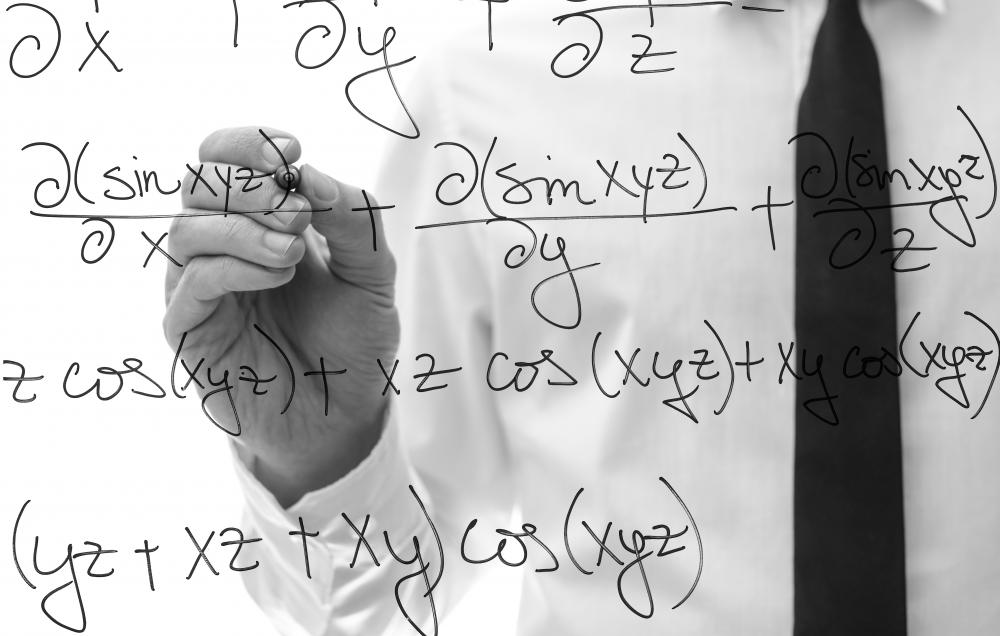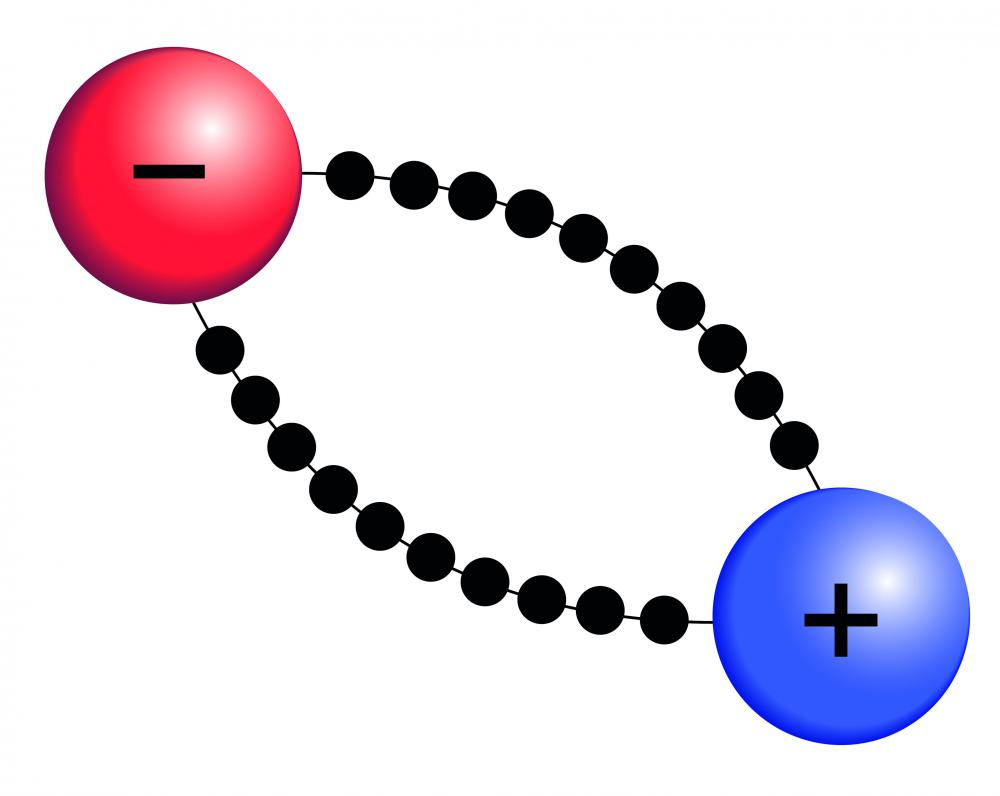What Is a Coulomb?

M.J. Casey
M.J. CaseyRobert Millikan won the 1923 Nobel prize for his work in measuring the charge of an electron.

A coulomb is a measure of electrical charge and is defined as the charge that flows with a constant current of one ampere (1 amp) during one second. The charge may be either positive or negative. It is equivalent to 6.241 x 1018 electrons. The idea of a coulomb is useful in electrochemistry and physics.

Properties in electricity are often compared to the physics of water in pipes or rivers. In this analogy, the amps, or amperage, is the rate of flow of water, and the coulomb is the amount of water transported during a given period of time. In everyday circumstances, most people concern themselves with the voltage an appliance requires or the wattage of a replacement light bulb. The actual charge flow or the coulombs used is only important when it is time to pay the electric bill.A coulomb is a measure of electrical charge and is defined as the charge that flows with a constant current of one ampere during one second.

An electrical charge is not the same thing as an electron. An electron is a subatomic particle that carries a charge. In a piece of metal, the electrons are fairly loosely held in place by the protons, the positively charged core of the atom. Once a potential or voltage is applied, the electrons shift in a uniform way, and charge is transferred as long as there is a potential difference between the ends of the metal, just as a river requires a vertical drop to flow.

As the charge of one coulomb is equivalent to the sum of the charges of 6.241 x 1018 electrons, the charge on one electron is the inverse: 1 divided by 6.241 x 1018 electrons per coulomb. The charge on one electron is 1.602 x 10-19 coulombs. Robert Millikan won the 1923 Nobel prize for his work in measuring the charge of an electron.

The most familiar transfer of charge occurs in a household electric circuit. A metal conductor, typically a copper wire, is used to transfer electricity from the house supply to a light. When a light switch is turned on, the light appears instantly. This happens because the charge is actually moving faster than the electrons. It also is moving in the opposite direction.

Some solids transfer charges as positively charged protons. Sometimes, the charge carriers of these solids are described as electron holes, or where electrons should be. Semiconductors used in electronics are often made of positive charge-carrying materials. In these materials, the charge is moving in the same direction as the charge carriers. Electrolyte solutions, solutions of dissolved minerals or other substances, carry charges in two directions, as the negatively charged ions will travel one way and the positively charged ions will travel the opposite.

Coulombs are used in calculations involving electrochemistry and by physicists studying electricity and magnetism. A common high school chemistry problem will inquire how much metal is deposited onto a substrate in a metal plating bath, given the amperage and length of time the current is applied. A physics problem using coulombs might be concerned with the efficiency of a photovoltaic panel, which converts photon energy to electricity.

You might also Like